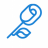# Using Fold with an adjustable end point

H

#### HawaiianIceberg

##### New member
I'm using a fold statement and want to have the "to" portion of the loop set to a dynamic number, as opposed to a static one. Like this, where BarsAgoForHigh is calculated previously using a different Fold statement

Def GetLowest = fold j = 0 to BarsAgoForHigh

In other words, BarsAgoForHigh is recalculated every bar, and the fold statement needs to loop that many times

If I set it to a static number, like this, it loops the desired 5 times:

Def GetLowest = fold j = 0 to 5

Here's more specifically what I'm doing. I'm looking for a large upward price swing, followed by price stabilization.

In this example, the stabilization period is 7 days, and the time-frame for the price swing up is 21. So price went up in days 1 through 21, then stabilized in week 4 (days 22-28).

This is the logic:

Starting with 7 days ago, Look back at the prior 21 days to see if there is a jump in price

def HighestHigh = Highest(High, 21); # Find the highest high from 7 bars ago back to 28 days ago
def LowestLow = Lowest(Low, 21); # Find the highest high from 7 bars ago back to 28 days ago

If there is a price jump, I use a fold loop to calculate how many bars back the highest high was (This variable is called BarsAgoForHigh)

def BarsAgoForHigh = fold i = 0 to 7 with t = 0
do
if high == HighestHigh then
(
t+i
)
else
(
t
);

Then, I use another Fold loop to evaluate what the lowest low is since that high point, which occurred BarsAgoForHigh bars ago. This is where the problem is

def GetLowest = fold j = 0 to BarsAgoForHigh with u = 10000
do
if getvalue(low, j+1) < getvalue(low, j) && getvalue(low, j+1) < u then
(
u*0+ getvalue(low, j+1)

)
else
(
u
);

When BarsAgoForHigh is static (for example, 5), it plots fine
When BarsAgoForHigh is dynamic (as outlined above) it doesn't plot anything (not even other simple plot commands in the study)

The function Lowest(low, BarsAgoForHigh) would be great, however it also does not allow a dynamic length (that's why I turned to Fold loops)

Thank you in advance. I'm just learning this language and find its simplicity can be difficult to work with

side note, is there a way to have an if statement that has multiple then commands lol?Last edited by a moderator:Scanner is using older candles than what is intended Questions 0